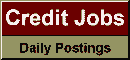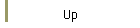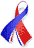DefaultRisk.com the web's biggest credit risk modeling resource.doi> search: A or B Export citation to:- HTML- Text (plain)- BibTeX- RIS- ReDIF

Model Risk in Copula Based Default Pricing Models

by Heinrich Gennheimer of the University of Zurich & NCCR FINRISK

November 2002

Abstract: This project identifies and quantifies two potential sources of model risk in the valuation of basket credit derivatives. The framework employed is that of a latent variable model with factor structure which enjoys a great popularity in the financial industry as well as in academia. Preliminary results indicate the prime importance of specifying the factor dependence structure beyond just estimating linear correlations for a Gaussian copula. Model risk is found to be substantial due to two reasons. Firstly, it is well known that the distribution functions of risk factors have lower tail-dependence. Derivatives prices are computed for models that are consistent with this fact. Their prices are shown to differ considerably, relative to the standard Gaussian model, for some products and dramatically for others. Secondly, the lack of convergence (even for very large numbers of simulation runs) for certain estimates that are functions of low probability events is another source of model risk. An efficient route to the solution of this second kind of model risk is the use of variance reduction techniques. Methods for variance reduction in copula based simulations are introduced and their efficiency is demonstrated. Moreover closed form solutions for the last-to default within a given portfolio are derived and worst case model risk which is often impossible to obtain by Monte Carlo simulation is calculated by means of these closed forms.

Books Referenced in this paper:  (what is this?)